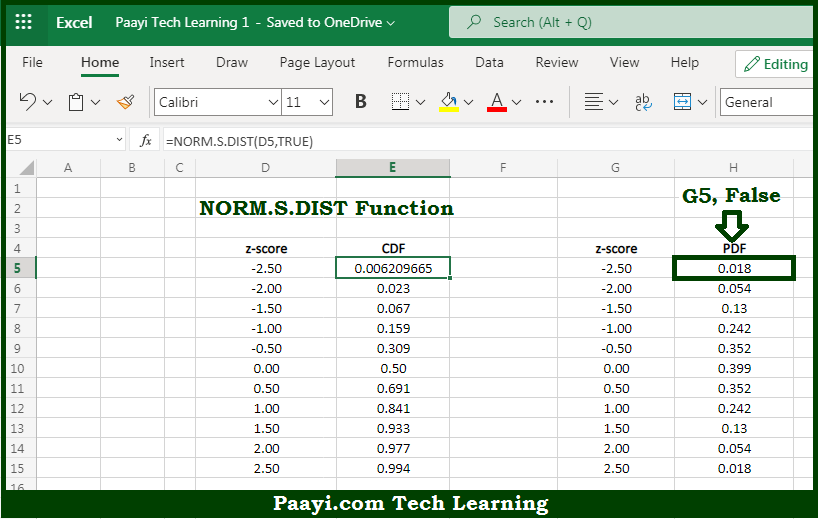# Learn How to Use Microsoft Excel NORM.S.DIST Function

Written by | 0 Comments | 583 Views

In this article, you will learn how to use Microsoft Excel NORM.S.DIST function and its prime function in Microsoft Excel. You will also get to know the Microsoft Excel NORM.S.DIST function return value and syntax with the help of some examples.

Microsoft Excel NORM.S.DIST Function

The main purpose of the Microsoft Excel NORM.S.DIST function is to get the standard normal CDF and PDF. That implies, with the help of NORM.S.DIST function you can able to return output for the standard normal cumulative distribution. which is CDF and the standard normal probability density function, that is PDF. So, with the help of NORM.S.DIST function, you can able to get the standard normal CDF and PDF.

Return Value of NORM.S.DIST Function

The return value will be the standard normal cumulative distribution function.

Syntax of NORM.S.DIST Function

=NORM.S.DIST(z, cumulative)

Where the arguments:

• z: This is the numeric z-score value.
• cumulative: This is the logical value that determines the form of the function.

## How to Use Microsoft Excel NORM.S.DIST Function?So we know that, Microsoft Excel NORM.S.DIST function you can able to get the standard normal CDF and PDF. That implies, with the help of NORM.S.DIST function you can able to return output for the standard normal cumulative distribution. which is CDF and the standard normal probability density function, that is PDF. So, with the help of NORM.S.DIST function, you can able to get the standard normal CDF and PDF.